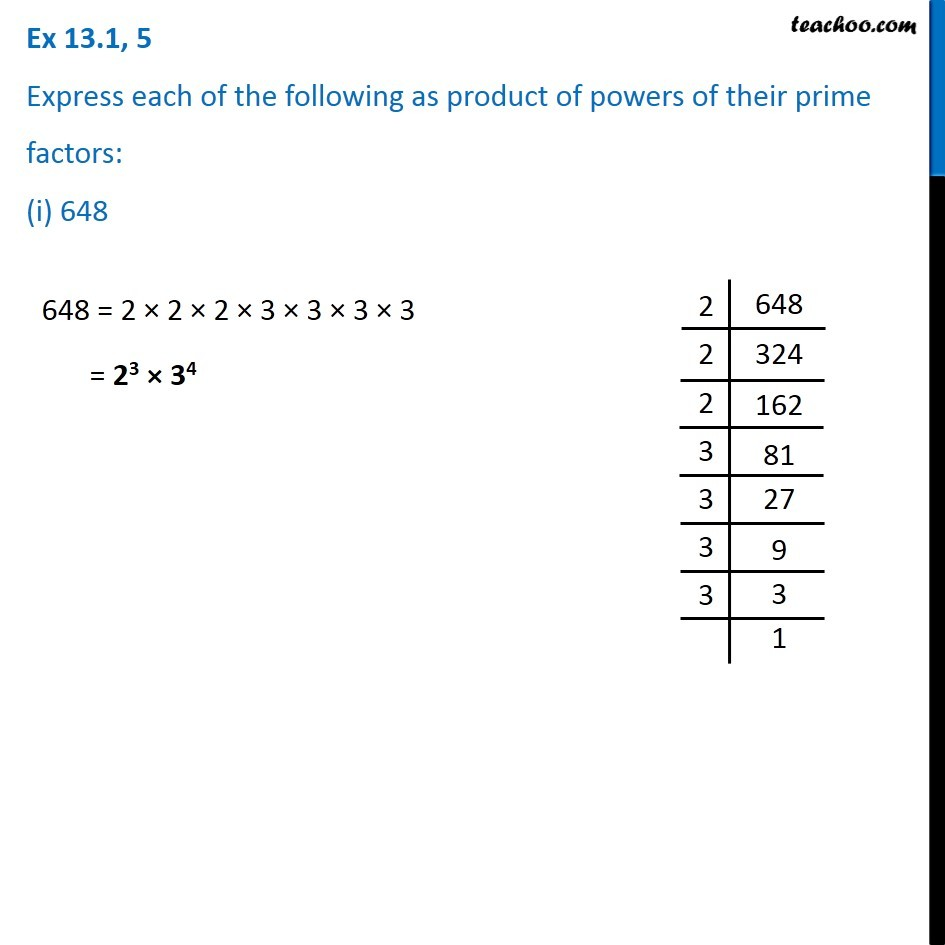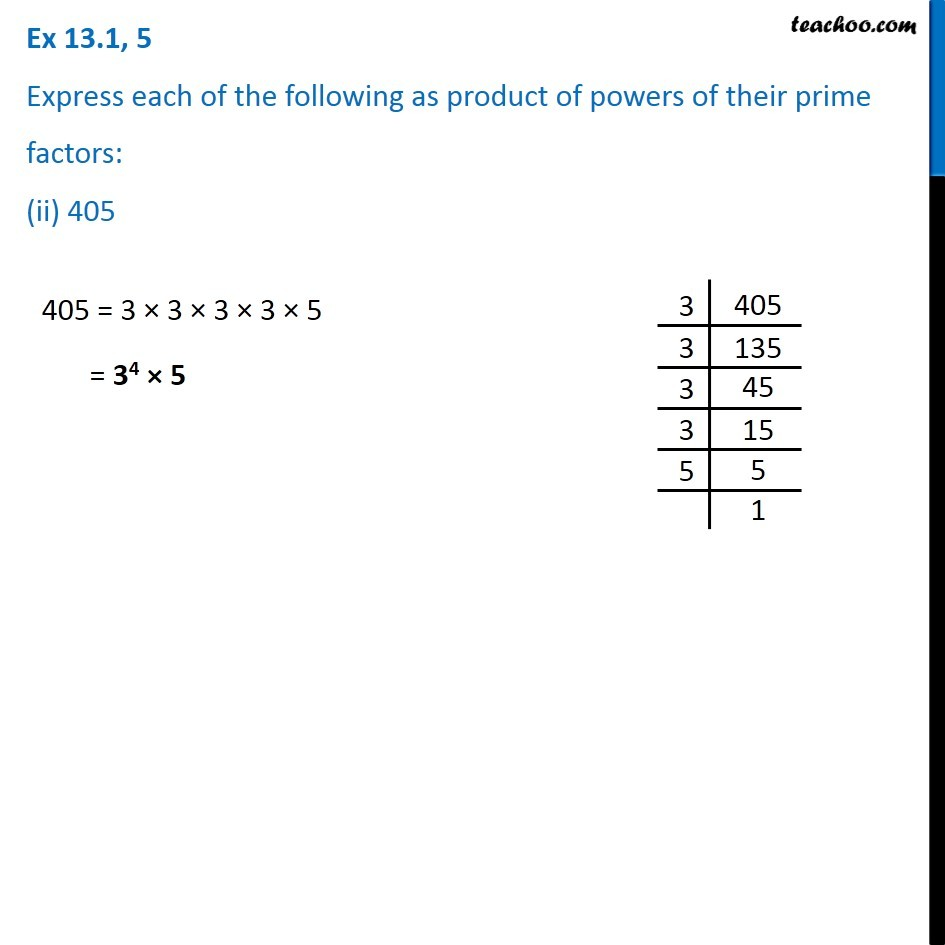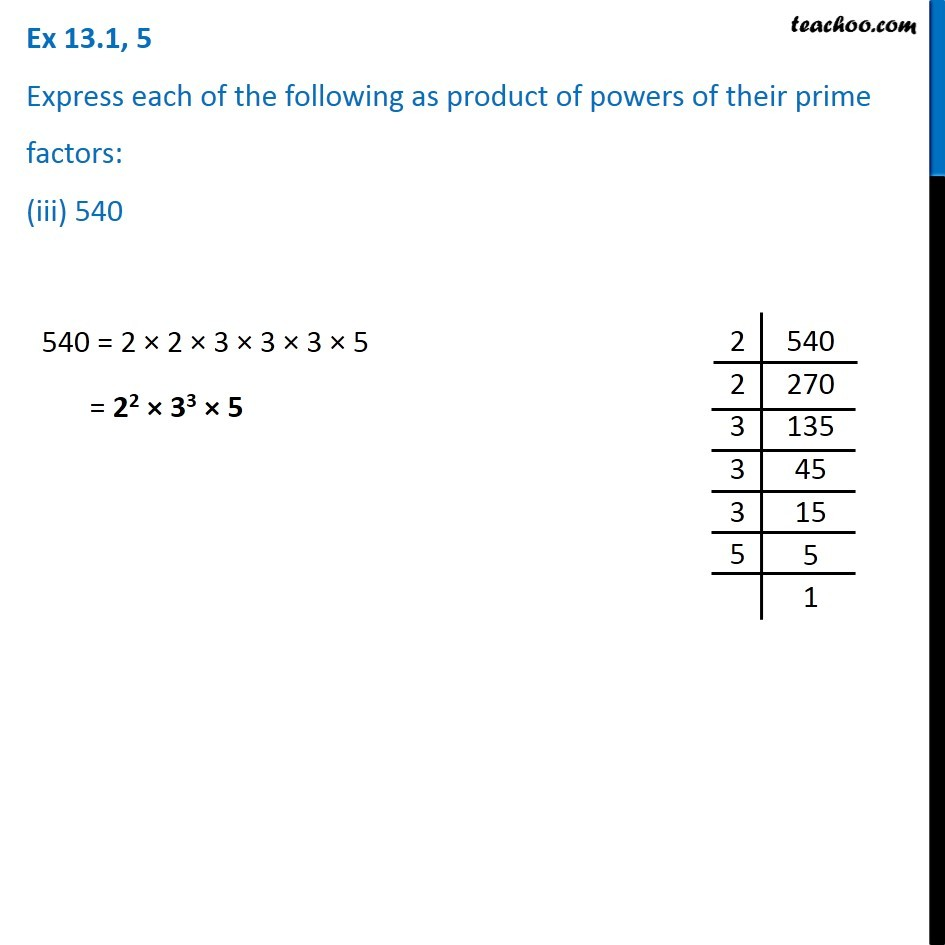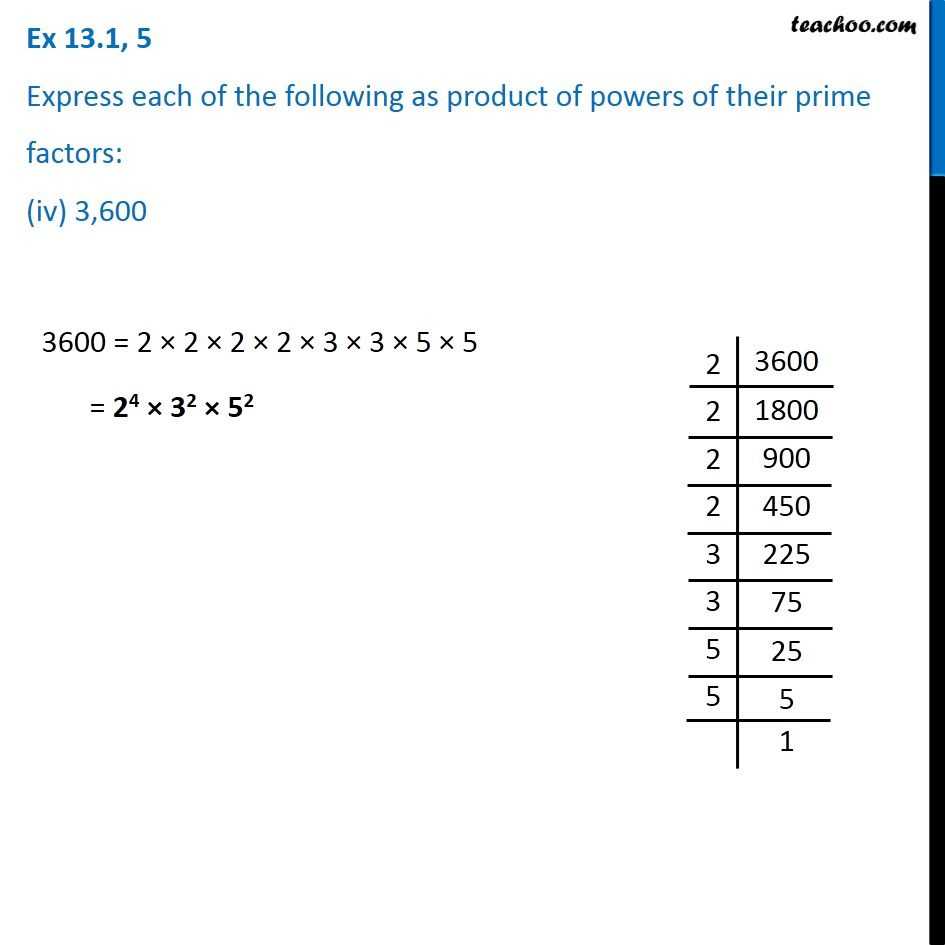Subscribe to our Youtube Channel - https://you.tube/teachoo

1. Chapter 13 Class 7 Exponents and Powers
2. Serial order wise
3. Ex 13.1

Transcript

Ex 13.1, 5 Express each of the following as product of powers of their prime factors: (i) 648 648 = 2 × 2 × 2 × 3 × 3 × 3 × 3 = 23 × 34 Ex 13.1, 5 Express each of the following as product of powers of their prime factors: (ii) 405 405 = 3 × 3 × 3 × 3 × 5 = 34 × 5 Ex 13.1, 5 Express each of the following as product of powers of their prime factors: (iii) 540 540 = 2 × 2 × 3 × 3 × 3 × 5 = 22 × 33 × 5 Ex 13.1, 5 Express each of the following as product of powers of their prime factors: (iv) 3,600 3600 = 2 × 2 × 2 × 2 × 3 × 3 × 5 × 5 = 24 × 32 × 52

Ex 13.1